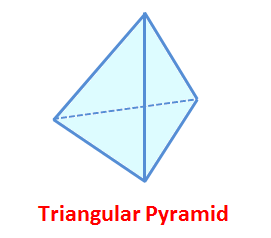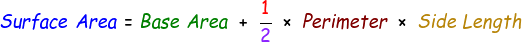# Surface Area of Triangular Pyramid Calculator

Triangular pyramids are the ones which possess a triangular base and it is also known as Tetrahedron. Below is the illustration of the triangular pyramid.We can calculate it's surface area using the below mentioned formula:Example: A triangular pyramid has a base area of 40Āmm, it's side length is 15Āmm and perimeter is 8. Calculate it's surface area.

Solution:

Given:Ā

Base Area = 40Āmm

Side Length = 15Āmm

Perimeter = 8

We know the formula, Surface Area of Triangular Pyramid = Base Area + 1/2 x Perimeter x Side Length

Ā= 40 + 1/2 x 8 x 15

Ā= 40 + 60

Ā= 100 mm2

Therefore, surface area for the given triangular pyramid = 100 mm2

Use our below online calculator to find surface area of triangular pyramids. Enter the required values and then click calculate to find the output.

 Base Area: Side Length: Perimeter: Surface Area of Triangular Pyramid:

Latest Calculator Release

Average Acceleration Calculator

Average acceleration is the object's change in speed for a specific given time period. ...

Free Fall Calculator

When an object falls into the ground due to planet's own gravitational force is known a...

Torque Calculator

Torque is nothing but a rotational force. In other words, the amount of force applied t...

Average Force Calculator

Average force can be explained as the amount of force exerted by the body moving at giv...

Angular Displacement Calculator

Angular displacement is the angle at which an object moves on a circular path. It is de...